Cube One
 To the Main Page    "Mathematische Basteleien"

What is Cube One ?
Cube One is a new cube puzzle invented by the graphic designer and artist Dieter A.W. Junker from Kassel / Germany.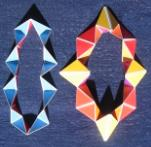The object is to build a cube with four chains of pyramids (kaleidocycles).

Drawings:+++>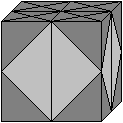Every kaleidocycle is a puzzle itself. The first chain leads to a tetrahedron, the second one to an octahedron.>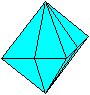>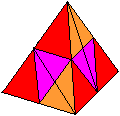I use the same colours  for my drawings as in the puzzle.

Investigation of the Octahedron Chain   top
Description
 ...... 16 congruent pyramids form the octahedron chain.  Two pyramids are symmetric to each other.

Formation of the pyramid red/blue
There is a pyramid at the corner of the cube, if you connect the centre points of three edges. If you lay a section through the diagonals of the cube, the pyramid at the corner is halved. This solid is the pyramid of the octahedron chain.
You can see the position in the following 3D-picture.Measurements of the pyramid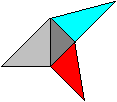Let "a" be the length of an edge of the cube. The grey basic triangle has the sides a/2 and sqrt(2)/4*a twice. The light grey triangle has the sides  sqrt(2)/2*a and a/2 twice. The blue triangle has the sides sqrt(2)/2*a, sqrt(2)/4*a, and sqrt(6)/4*a. The red triangle has the sides a/2, sqrt(2)/4*a, and sqrt(6)/4*a.

Volume
The pyramid has the grey triangle as basic triangle and the height a/2.
The volume V1' is (1/3)*[(1/2)(a/2)*(a/2)/2]*(a/2)=a³/96.
The octahedron chain has the volume V1= 16V1' =16a³/96=a³/6.

Investigation of the Tetrahedron Chain     top
Description
 ...... 16 congruent pyramids form the tetrahedron chain There are two kinds of pyramids, the pyramid violet/yellow and the pyramid red/orange. Two pyramids are symmetrical to each other.

Formation of the first pyramid (violet/yellow)
You get a pyramid if you connect certain centres of the edges.
You can see this in a 3d-picture.Measurements of the pyramid
 ...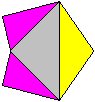... Let "a" be the length of an edge of the cube. The grey triangle has the sides "a" and sqrt(2)/2*a twice. The yellow triangle has the sides "a" and sqrt(6)/4*a twice. The violet triangles are congruent and have the sides sqrt(2)/2*a, sqrt(2)/4*a, and sqrt(6)/4*a.

Volume
The pyramid has the yellow triangle as basic triangle and the height sqrt(6)/4*a.
The volume V21'  is (1/3)*[(a/2)*(sqrt(2)/4*a]*(sqrt(2)/4*a)=a³/48.

Formation of the second pyramid (red/orange)
You get a pyramid if you connect certain centres of the edges.
You can see this in a 3d-picture.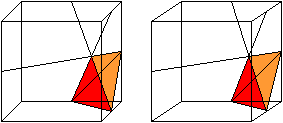Measurements of the pyramid
 ...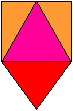... Let "a" be the length of an edge of the cube. The triangle in the centre is equilateral and has the sides sqrt(2)/2*a. The red triangle below has the sides sqrt(2)/2*a and sqrt(6)/4*a twice. The violet triangles are congruent and have the sides sqrt(2)/2*a, sqrt(2)/4*a, and sqrt(6)/4*a.

Volume
The pyramid has the red triangle as basic triangle and the height sqrt(2)/4*a.
The volume V22' is (1/3)*[(a/2)*(sqrt(2)/4*a]*(sqrt(2)/4*a)=a³/48.

Volume of the tetrahedron chain
Eight pyramids violet/yellow and eight pyramids red/orange form the tetrahedron chain.
With that the volume is V2=8*V21' + 8V22' = 8a³/48+8a³/48=a³/3.

Division of the cubeV/6V/6V/3V/3

Solution of Cube One  top
First step
 ...... You put the octahedron chain on a plane as shown on the left and push together the pair of pyramids so that the red faces touch each other.
You get an opened octahedron as shown in the next 3d picture.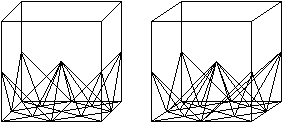transparent

You put this body in the transparent plastic cube, which belongs to this puzzle.Second step
 ...... You put the tetrahedron chain on the desk as shown on the left.  You must form the "crown". In the beginning it is slightly difficult.  Look at the yellow triangles. The four triangles at the top and below become the outer edges of the crown. The yellow triangles on the right and left approach inside. You must turn the chain. This is only possible with light power.transparent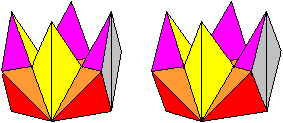non-transparent and coloured

Put the crown into the cube on the octahedron chain.Third step
Form a second crown from the tetrahedron chain and put it on the first one upside down.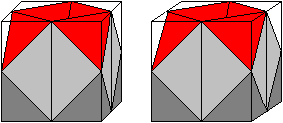Fourth step
Close the cube with a second opened octahedron.Second solution
There is a second solution as the inventor tells. This solved cube has this appearance.You find more about kaleidocycles on my homepage: Kaleidocycles (German/English) The Shinsei Miracle  (German only)

Cube One on the Internet   top

Dieter A.W. Junker
Flyping Games   (German/English)

Feedback: Email address on my main page